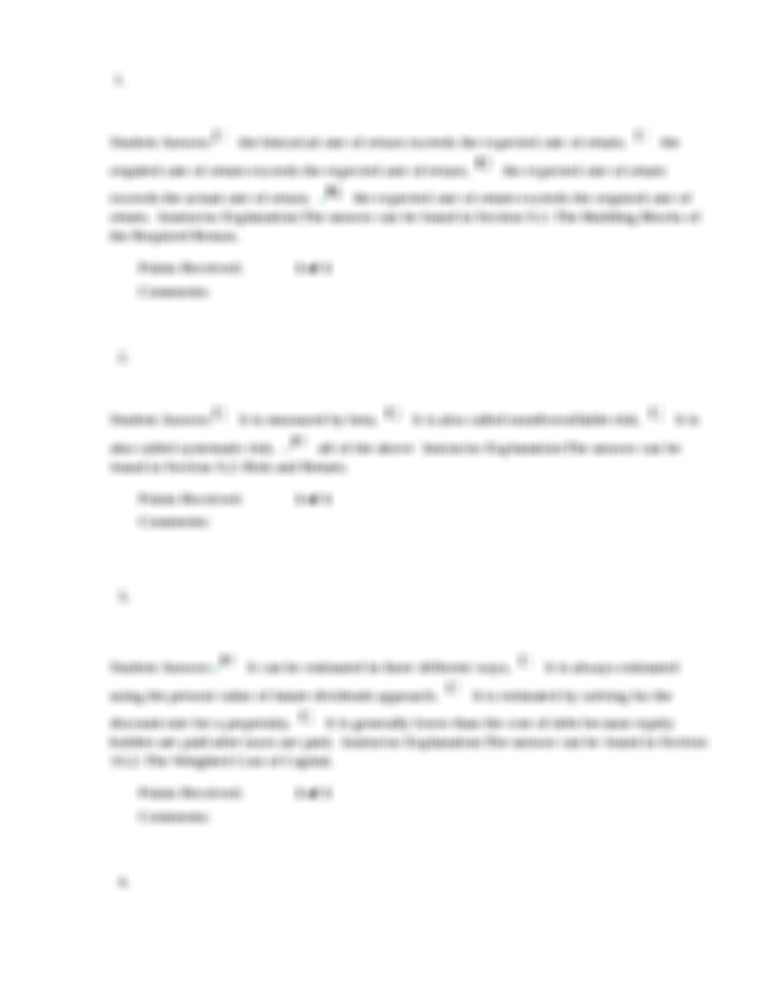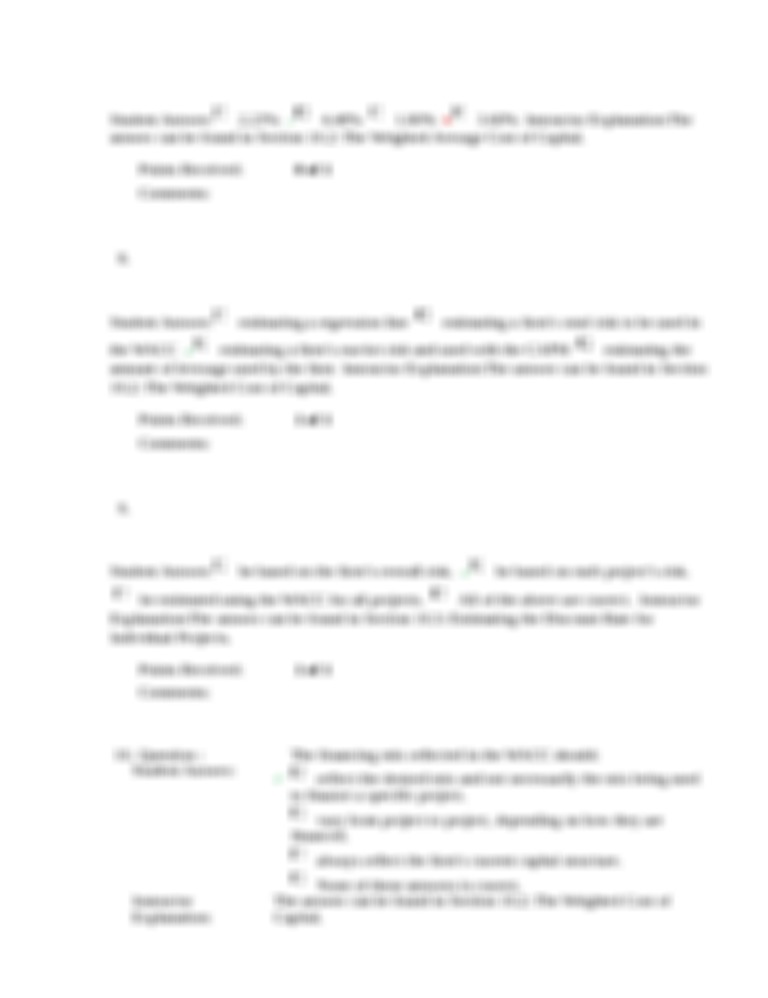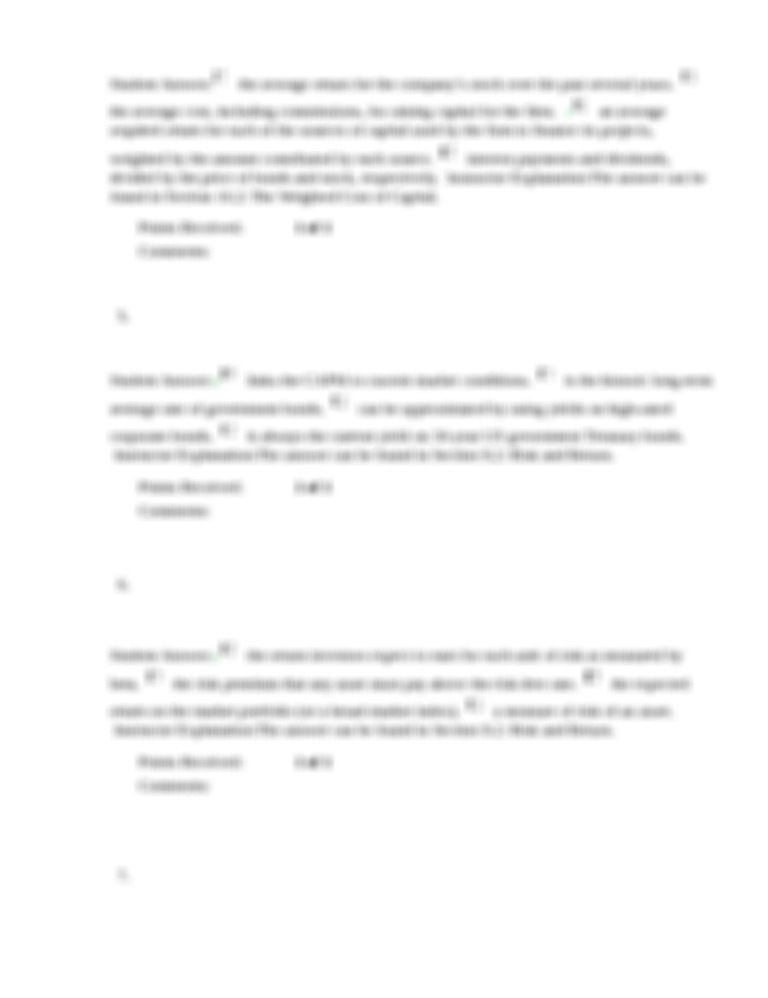Back to Search
Ashford
Principles of finance
week 4
BUS 401 Week 4 Quiz

# BUS 401 Week 4 Quiz

Short description

. Question : Investors will make an investment if: 2. Question : Which of the following is true regarding market risk? 3. Question : Which of the following statements regarding the cost of equity is true? 4. Question : The weighted average cost of capital is: 5. Question : In the Capital Asset Pricing Model, the risk-free rate: 6. Question : In the Capital Asset Pricing Model, the market risk premium can be thought of as: 7. Question : A bond pays semiannual coupon payments of \$30 each. It matures in 20 years and is selling for \$1,200. What is the firm’s cost of debt if the bond’s par value is \$1,000? (Don’t forget this is a semiannual coupon.) 8. Question : Which of the following is beta is used for? 9. Question : The discount rate used in project evaluation should: 10. Question : The financing mix reflected in the WACC should:

Document previewBack to Search